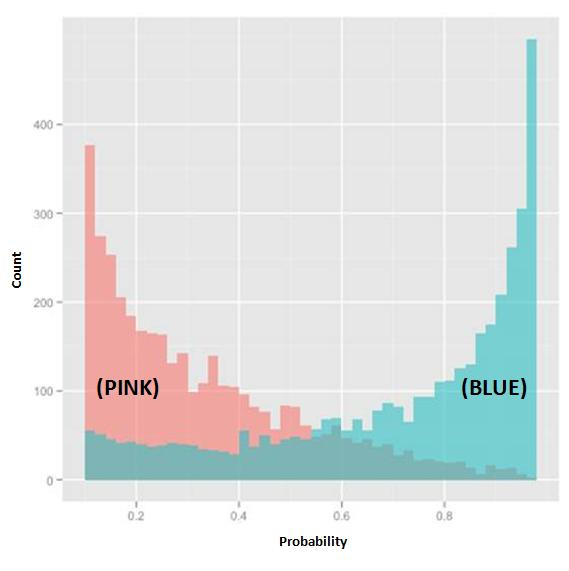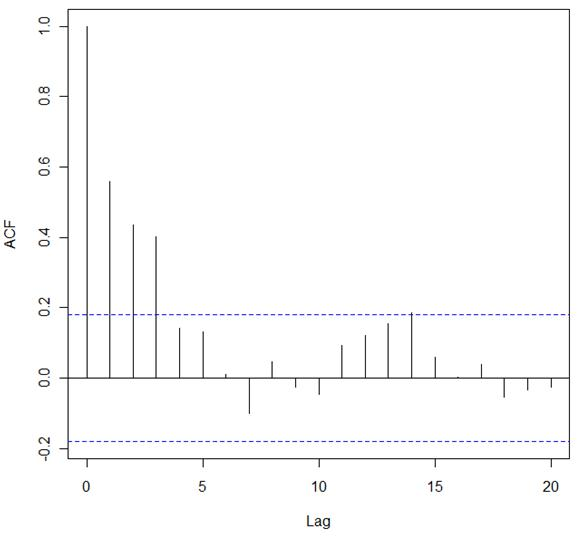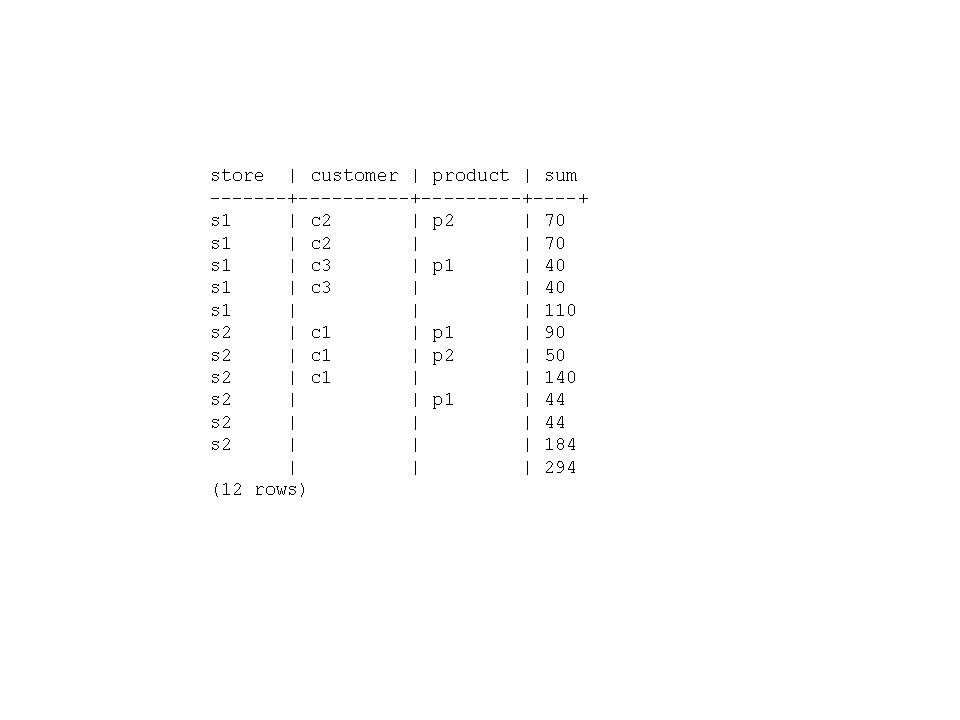# Data Science Associate Exam v7.0

Page:    1 / 14
Exam contains 206 questions

You are building a logistic regression model to predict whether a tax filer will be audited within the next two years. Your training set population is 1000 filers. The audit rate in your training data is 4.2%. What is the sum of the probabilities that the model assigns to all the filers in your training set that have been audited?

• A. 42.0
• B. 4.2
• C. 0.42
• D. 0.042

You are using the Apriori algorithm to determine the likelihood that a person who owns a home has a good credit score. You have determined that the confidence for the rules used in the algorithm is > 75%. You calculate lift = 1.011 for the rule, "People with good credit are homeowners". What can you determine from the lift calculation?

• A. Support for the association is low
• B. Leverage of the rules is low
• C. The rule is coincidental
• D. The rule is true

In a Student's t-test, what is the meaning of the p-value?

• A. it is the area under the appropriate tails of the Student's distribution
• B. it is the "power" of the Student's t-test
• C. it is the mean of the distribution for the null hypothesis
• D. it is the mean of the distribution for the alternate hypothesis

In the MapReduce framework, what is the purpose of the Map Function?

• A. It processes the input and generates key-value pairs
• B. It collects the output of the Reduce function
• C. It sorts the results of the Reduce function
• D. It breaks the input into smaller components and distributes to other nodes in the cluster

What is a core deliverable at the end of the analytic project?

• A. An implemented database design
• B. A whitepaper describing the project and the implementation
• C. A presentation for project sponsors
• D. The training materials

Consider a database with 4 transactions:
Transaction 4: {cheese, soda, juice}
The minimum support is 25%. Which rule has a confidence equal to 50%?

• A. {bread, milk} => {cheese}
• C. {juice} => {soda}

Which data asset is an example of semi-structured data?

• A. XML data file
• B. Database table
• C. Webserver log
• D. News article

Refer to the exhibit.In the exhibit, the x-axis represents the derived probability of a borrower defaulting on a loan. Also in the exhibit, the pink represents borrowers that are known to have not defaulted on their loan, and the blue represents borrowers that are known to have defaulted on their loan.
Which analytical method could produce the probabilities needed to build this exhibit?

• A. Logistic Regression
• B. Linear Regression
• C. Discriminant Analysis
• D. Association Rules

In linear regression modeling, which action can be taken to improve the linearity of the relationship between the dependent and independent variables?

• A. Apply a transformation to a variable
• B. Use a different statistical package
• C. Calculate the R-Squared value
• D. Change the units of measurement on the independent variable

A Data Scientist is assigned to build a model from a reporting data warehouse. The warehouse contains data collected from many sources and transformed through a complex, multi-stage ETL process. What is a concern the data scientist should have about the data?

• A. It is too processed
• B. It is not structured
• C. It is not normalized
• D. It is too centralized

The average purchase size from your online sales site is \$17, 200. The customer experience team believes a certain adjustment of the website will increase sales. A pilot study on a few hundred customers showed an increase in average purchase size of \$1.47, with a significance level of p=0.1.
The team runs a larger study, of a few thousand customers. The second study shows an increased average purchase size of \$0.74, with a significance level of 0.03. What is your assessment of this study?

• A. The change in purchase size is not practically important, and the good p-value of the second study is probably a result of the large study size.
• B. The change in purchase size is small, but may aggregate up to a large increase in profits over the entire customer base.
• C. The difference in the change in purchase size between the two studies is troubling; The team should run another, larger study.
• D. The p-value of the second study shows a statistically significant change in purchase size. The new website is an improvement.

You have been assigned to run a logistic regression model for each of 100 countries, and all the data is currently stored in a PostgreSQL database. Which tool/library would you use to produce these models with the least effort?

• B. Mahout
• C. RStudio
• D. HBase

Refer to the exhibit.In the exhibit, a correlogram is provided based on an autocorrelation analysis of a sample dataset.
What can you conclude from only this exhibit?

• A. There is significant autocorrelation through lag 3
• B. There is no structure left to model in the data
• C. Lag 7 has a significant negative autocorrelation
• D. Differencing is required before proceeding with any analysis

Consider a database with 4 transactions:
Transaction 4: {cheese, soda, juice}
You decide to run the association rules algorithm where minimum support is 50%. Which rule has a confidence at least 50%?

• B. {juice} => {cheese}
• C. {milk} => {soda}
• D. {soda} => {milk}

Refer to the Exhibit.You are working on creating an OLAP query that outputs several rows of with summary rows of subtotals and grand totals in addition to regular rows that may contain NULL as shown in the exhibit. Which function can you use in your query to distinguish the row from a regular row to a subtotal row?

• A. GROUPING
• B. RANK
• C. GROUP_ID
• D. ROLLUP